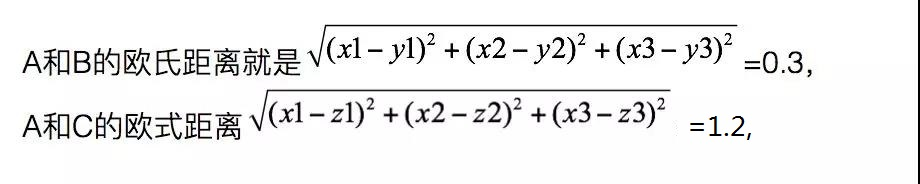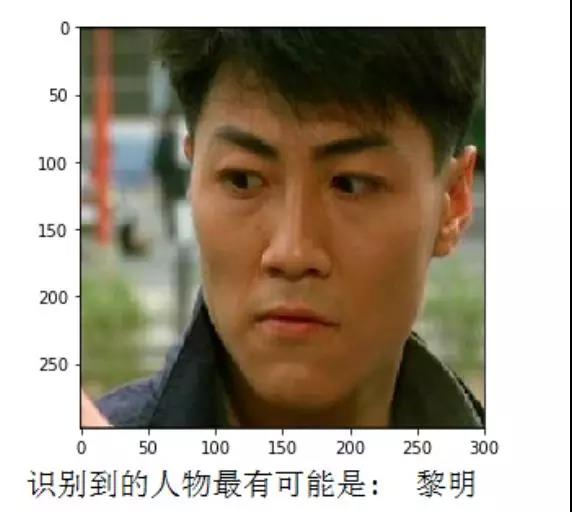# 用Python实现一个简单的——人脸相似度对比

1、预先导入所需要的人脸识别模型；

2、遍历循环识别文件夹里面的图片，让模型“记住”人物的样子；

3、输入一张新的图像，与前一步文件夹里面的图片比对，返回最接近的结果。

1、模块：os,dlib,glob,numpy；

2、模型：人脸关键点检测器，人脸识别模型。

shape_predictor.dat这个是为了检测人脸的关键点，比如眼睛，嘴巴等等；dlib_face_recognition.dat是在前面检测关键点的基础上，生成人脸的特征值。

import os,dlib,glob,numpy
from skimage import io

# 人脸关键点检测器
predictor_path = "shape_predictor.dat"
# 人脸识别模型、提取特征值
face_rec_model_path = "dlib_face_recognition.dat"
# 训练图像文件夹
faces_folder_path ='train_images'

# 加载模型
detector = dlib.get_frontal_face_detector()
sp = dlib.shape_predictor(predictor_path)
facerec = dlib.face_recognition_model_v1(face_rec_model_path)

candidate = []         # 存放训练集人物名字
descriptors = []       #存放训练集人物特征列表

for f in glob.glob(os.path.join(faces_folder_path,"*.jpg")):
print("正在处理: {}".format(f))
candidate.append(f.split('\\')[-1].split('.'))
# 人脸检测
dets = detector(img, 1)
for k, d in enumerate(dets):
shape = sp(img, d)
# 提取特征
face_descriptor = facerec.compute_face_descriptor(img, shape)
v = numpy.array(face_descriptor)
descriptors.append(v)

print('识别训练完毕！')try:
##    test_path=input('请输入要检测的图片的路径（记得加后缀哦）:')
dets = detector(img, 1)
except:
print('输入路径有误，请检查！')

dist = []
for k, d in enumerate(dets):
shape = sp(img, d)
face_descriptor = facerec.compute_face_descriptor(img, shape)
d_test = numpy.array(face_descriptor)
for i in descriptors:                #计算距离
dist_ = numpy.linalg.norm(i-d_test)
dist.append(dist_)

# 训练集人物和距离组成一个字典
c_d = dict(zip(candidate,dist))
cd_sorted = sorted(c_d.items(), key=lambda d:d)
print ("识别到的人物最有可能是: ",cd_sorted){'刘亦菲': 0.5269014581137407,

'刘诗诗': 0.4779630331578229,

'唐艺昕': 0.45967444611419184,

'杨幂': 0.4753850256188804,

'迪丽热巴': 0.5730399094704894,

'郑秀妍': 0.40740137304879187,

'郑秀晶': 0.45325515192940385,

'郭富城': 0.7624925709626963,

'黎明': 0.5925473299225084}